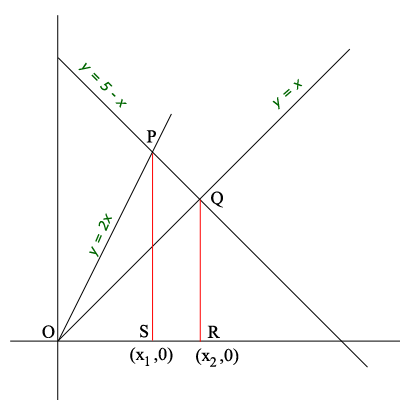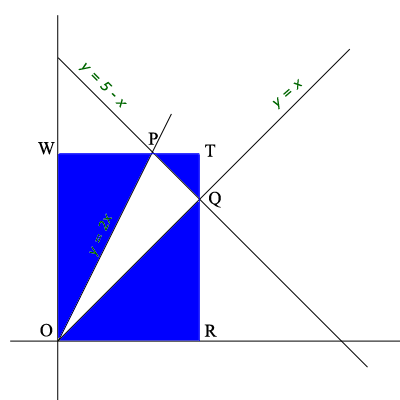SEARCH HOMEMath Central Quandaries & QueriesQuestion from sidra, a student: find area bounded by functions: y=x y=2x and y=5-xHi Sidra,

I think This is for a calculus class so you are expected to use integration. Here is my diagram.Solve the equation $y = 5 - x$ with both of the equations $y = x$ and $y = 2x$ to determine the coordinates $(x_1, y_1)$ and $(x_2, y_2)$ of $P$ and $Q$ respectively. The area of the triangle $POQ$ is the area of the triangle $POS$ plus the area of the trapezoid $PSRQ$ minus the area of the triangle $QOR.$ Each of these can be found using integration.

If I were to find this area for anything but a calculus class I certainly wouldn't use integration. First of all the line $y = x$ has slope 1 and the line $y = 5 - x$ has slope -1 and thus these line are perpendicular. Thus the triangle $POQ$ is a right triangle and thus if you find the lengths of $OQ$ and $QP$ you can calculate that area of the triangle using half the base times the height.

Even easier is to surround the triangle $POQ$ with the rectangle $TWOR.$The area of the triangle $POQ$ is the area of the rectangle minus the sum of the areas of the three blue triangles. Each of these triangles is a right triangle with sides parallel to the coordinate axes and its area is easy to calculate.

PennyMath Central is supported by the University of Regina and The Pacific Institute for the Mathematical Sciences.